# Chemistry (Math in Disguise of Science)

Flashcard maker : Lily Taylor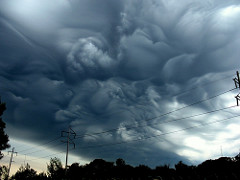Calculate the mass in grams 1.25 moles of aluminum chloride.
1. Add the atomic mass, multiply them if they have a little number.
2. Multiply the number they gave you over 1 GRAM and the number you got from adding the masses over 1 MOLE.
EX
Al-26.98
Cl-(3)35.45
133.33

1.25 mol/1 g X 133.33 g/1 mol= 166.62 g /mol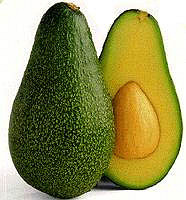Calculate the number of molecules present in 4.75 g of phosphine , PH3(little 3).
1. Add the atomic mass, multiply them if they have a little number.
2. Multiply the number they gave you over 1 MOLECULE and the AVAGOTES number over the number you got.

EX
P-30.97
H-(3)1.0079
33.99 g

4.75g/1 Molecules X 6.22×10^23/33.99 g = 8.42×10^23 MoleculesCalculate the percent by mass of Methane CH4(little 4).
1. Add the atomic mass, multiply them if they have a little number.
2. Atomic mass g over the number you got.
3. Times 100

EX
C-12.01
H-(4)1.0079
16.04 g

C- 12.01gC/16.04g X 100= 75%
H- 4.03gH/16.04g X 100=25%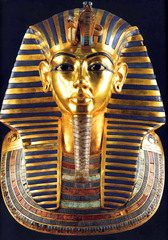A compound was analyzed and found to contain the following .
Baruim-89.59%
Oxygen-10.44%
Determine the empirical formula of it.
1. Change the percent to grams.
2. Put the number they gave you over 1 MOLE and multiply it by 1 MOLE over its atomic mass.
3. Put the number you got over the smallest number.
4. The number you now have is the subscript.

EX
Ba-89.59%=89.59g
O-10.44%=10.44g

89.59g Ba/ 1 mol X 1 mol/ 137.3 g = 0.6253 mol Ba
10.44g O/ 1 mol X 1 mol/16.00 g = 0.6253 mol O

0.6253 mol Ba/0.6253 mol =1 mol Ba
0.6253 mol O/0.6253 mol = 1 mol O
=
BaO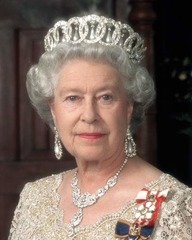An oxide of aluminum if formed by the reaction of 4.15 g of aluminum with 3.692 g of oxygen. Calculate the empirical formula for this compound.
1. Put the number they gave you over 1 mole times 1 mol over the atomic mass.
2. Divide both by the smallest number.
3. If you don’t get a full number , than multiply ALL till you do.

EX
Al- 4.151g Al/1 mol X 1 mol/26.98 g Al = .1538
O- 3.692g O/1 mol X 1 mol/ 16 G O= .23075

.1538/ .1538 = 1 X 2= 2
.23075/.1538=1.5 X 2=3
=Al2O3 ( Little 2 & Little 3)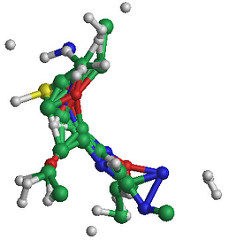A compound with the empirical formula CH4O (little 4) was formed in a subsequent experiment to have a molar mass of apprimatldy 192 g. What is the molecule formula of the compound?
1. Add the atomic mass, multiply them if they have a little number.
2. Put the number they gave you over the number you got.
3. If you got number that info full multiply it till you do.

EX
C-12.01
H-(4)1.008
O-15.9994
32.0414

192g/32.0414g = 5.99= 6
C6H24O6 (little 4, little 24, little 6)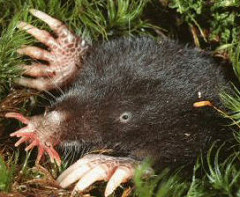Calculate the number of moles in 6.22 g of Aluminum.
1. Put the number they gave you over 1 mole and times it by 1 mole over the atomic mass

EX
6.22 g Al/ 1 mol X 1 mol/ 26.98 g Al = 0.23 g/mol Al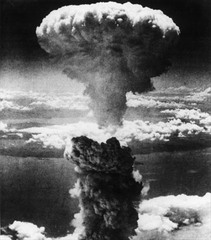Calculate the molar mass for magnesium nitride, Mg(NO2)2 ( little 2 ,little 2).
1. Add the atomic mass, multiply them if they have a little number.

EX
Mg-24.305
N-(2)14.00674
O-(4)15.9994
116.31